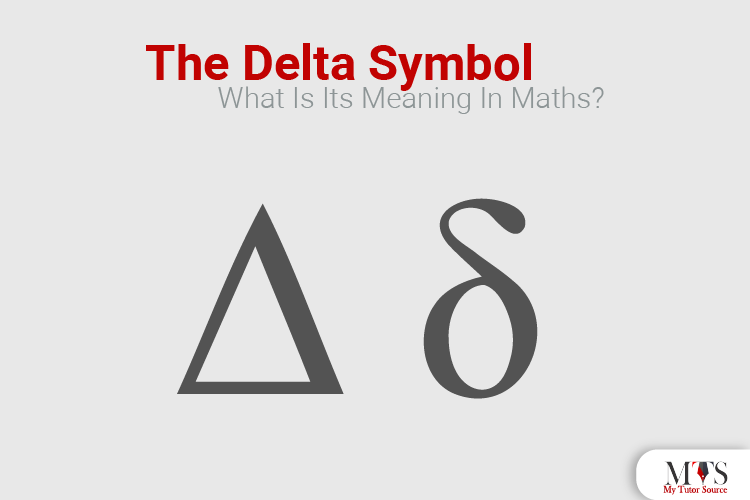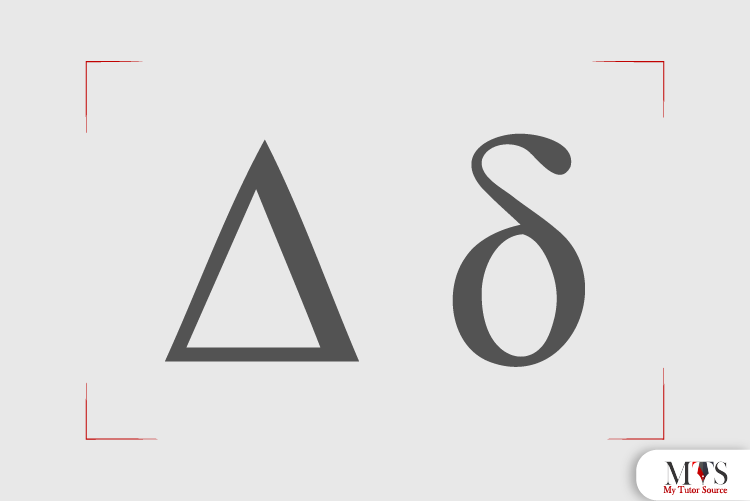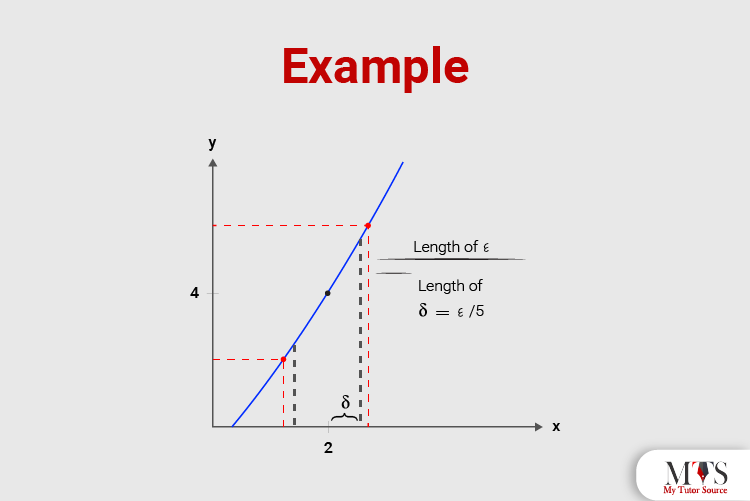# The Delta Symbol: What Is Its Meaning in Maths?

• Published On:Mathematics has undergone a lot of development over the years, and with each progress, new things and concepts were added. Mathematicians started introducing new symbols to represent different functions, equations, sets, and numbers.

However, have you noticed that most of the symbols we see in Mathematics are made out of Greek letters and alphabets? Well, that is because Greek was a very common language back then, and most scholars found Greek an easy and understandable choice for symbols.

With that being said, you have most probably heard and seen the Greek symbol‘ Delta’ in Mathematics, right? In this post, we are going to explain what the delta symbol means, what it represents, and how it can symbolize multiple concepts. Keep Reading!## What does Delta Symbol mean?

Delta is the fourth letter of the Greek Alphabet, and it was derived from the Phoenician letter dalet. The delta symbol is widely used in Mathematics for multiple and significant concepts. In Mathematics, the Delta symbol is used to represent sets, equations, numbers, and functions.

## What Concepts does Delta Symbolize?

Here is a detailed list of concepts that the delta symbol represents in Mathematics:

### 1. Change

Delts in the upper-case form ( Δ ) usually represent ‘change’ or ‘the change’ in Mathematics. Let’s take an example; if we have a variable ‘x’ that stands for the movement of an object, Δx would mean ‘the change’ in the movement. This symbolization of delta is widely used by scientists in word problems of Mathematics, Engineering, Physics, and Chemistry.

### 2. Discriminant

In Algebra, the upper-case delta ( Δ ) is used to represent the discriminant of a polynomial equation. However, the polynomial equation should also always be a quadratic equation. For example, if we have a quadratic equation, ax2 + bx = c, then its discriminant would be ‘b2 - 4ac’, right? However, its representation would be  Δ = b2 - 4ac.

A discriminant helps provide information about the quadratic roots. The most important of them all is that a quadratic can have two real roots, two complex roots, or one real root. However, that totally depends on the value of Δ.

### 3. Geometry and Angles

The lower-case delta symbol ( δ ) is also widely used in geometry to represent angles in geometric shapes. This is because the roots and origin of Geometry are from Euclid in ancient Greece. Back then, mathematicians used to mark their angles with Greek letters. Here is a visual representation of how lower-case delta ( δ ) is used in geometry and angles:### 4. Partial Derivatives

The derivative of a function is known as the measure of infinitesimal changes in any one of its variables. A derivative is represented by the roman letter ‘d’. However, partial derivatives are a little different from regular derivatives. Its function contains multiple variables but only one variable is considered; the rest remain fixed. Partial derivatives are also represented by a lower-case delta ( δ ), and the equation of its functions will look like this: δf over δx.

### 5. Kronecker Delta (Lowercase Delta)

The lower-case delta ( δ ) is also widely used in Advanced Mathematics by the name of Kronecker Delta. It helps to represent the relationship between two integral variables; this will be 1 if two variables are equal, and 0 if no variables are equal.

Also, a Kronecker delta (Lowercase Delta) denotes the change of values of variables in calculus.

## Final Words

Now that you have gone through the entire, we hope you understand the usage and importance of the Delta in various fields of Mathematics and Science. If you still have any uncertainties and confusion, visit our tutor's page and book a session with one of our top-rated and high-qualifies Mathematics tutors. They will clear all your concepts with their expertise and professional teaching skills.

### Find Top Tutors in Your AreaAustin has 10+ years of experience in teaching. He has researched on thousands of students-related topics, issues, and concerns. You will often find him writing about the common concerns of students, their nutrition, and what is beneficial for their academics and health both.